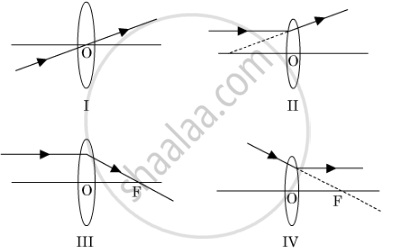# A student has obtained a magnified image of a flame on a screen using a convex lens. To draw the corresponding ray diagram to show the image formation, which of the following two rays whose paths after refraction are shown, should h - Science

A student has obtained a magnified image of a flame on a screen using a convex lens. To draw the corresponding ray diagram to show the image formation, which of the following two rays whose paths after refraction are shown, should he select ?(A) I and II

(B) II and III

(C) III and IV

(D) I and III

#### Solution

A convex lens converges the refracting ray. Therefore, rays I and III represent the path of the refracting ray from a convex lens(converging lens). As rays II and IV show the refracting ray being diverged, the ray diagrams are incorrect.

Hence, the correct option is D.

Concept: Convex Lens
Is there an error in this question or solution?